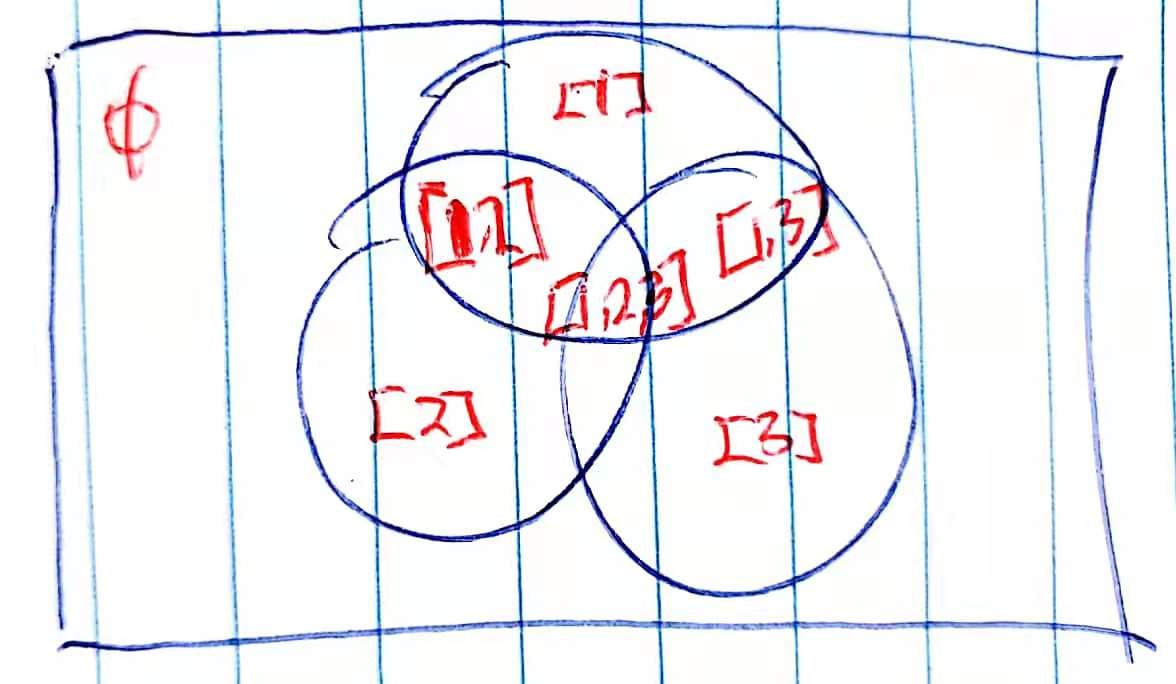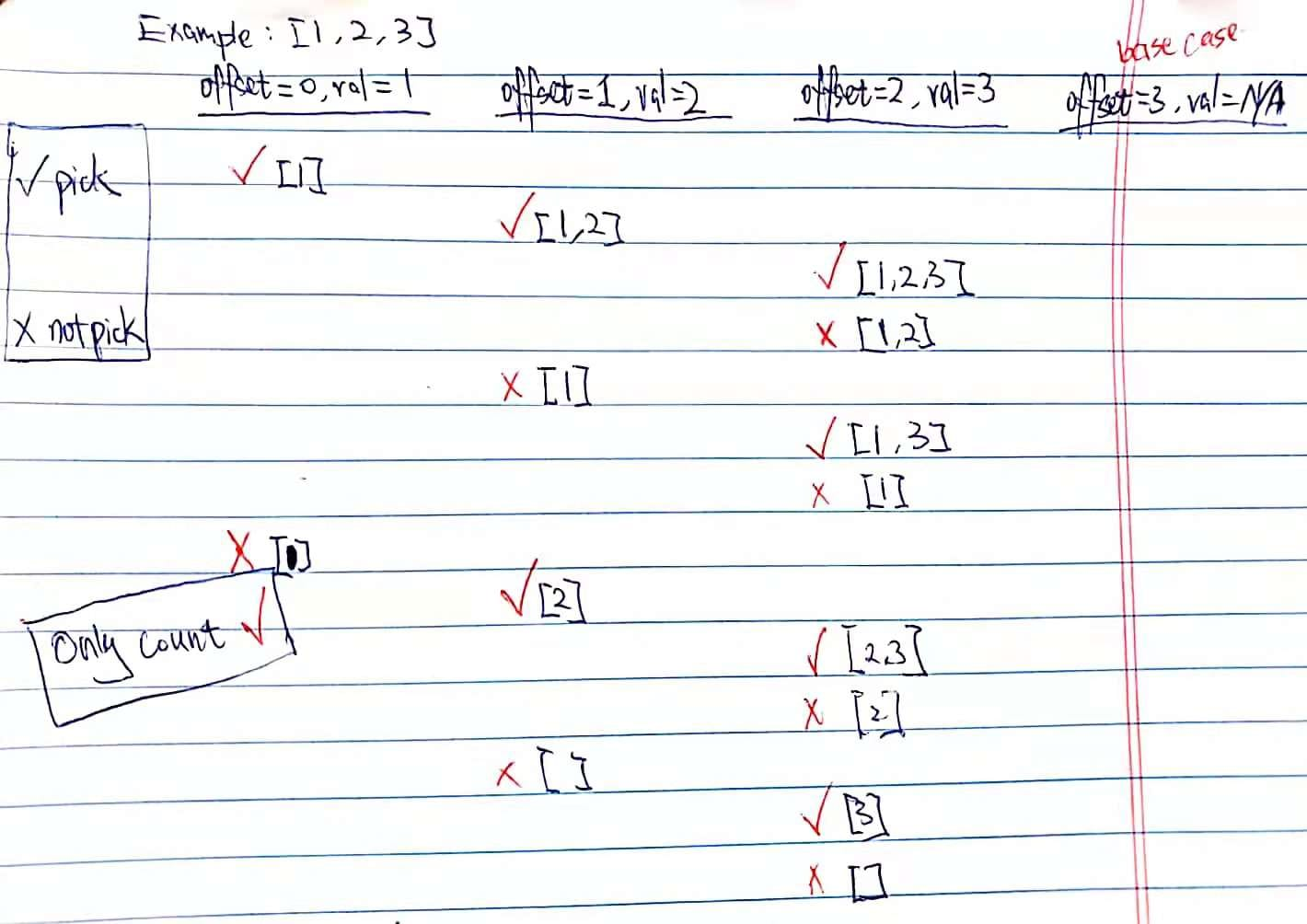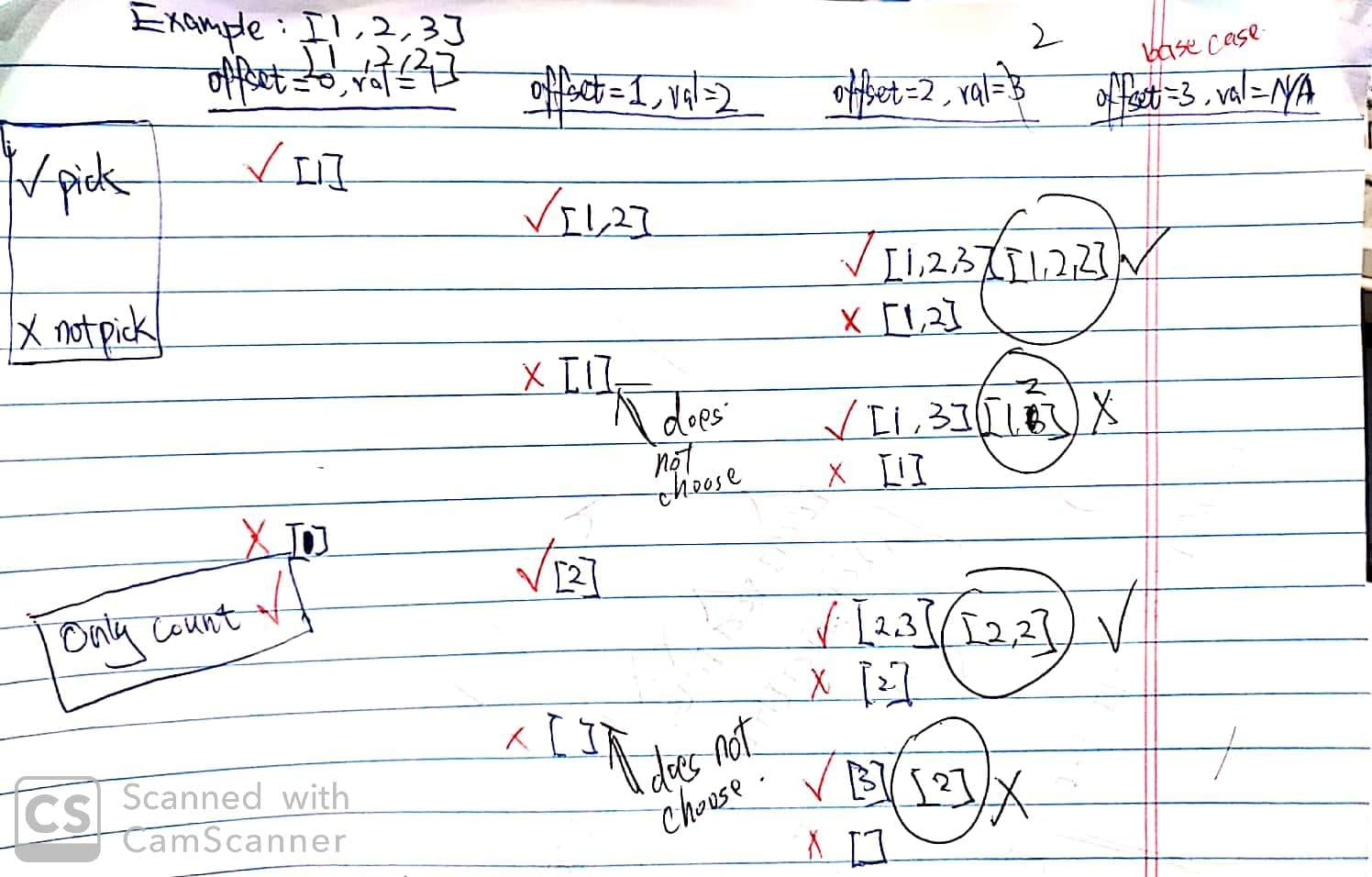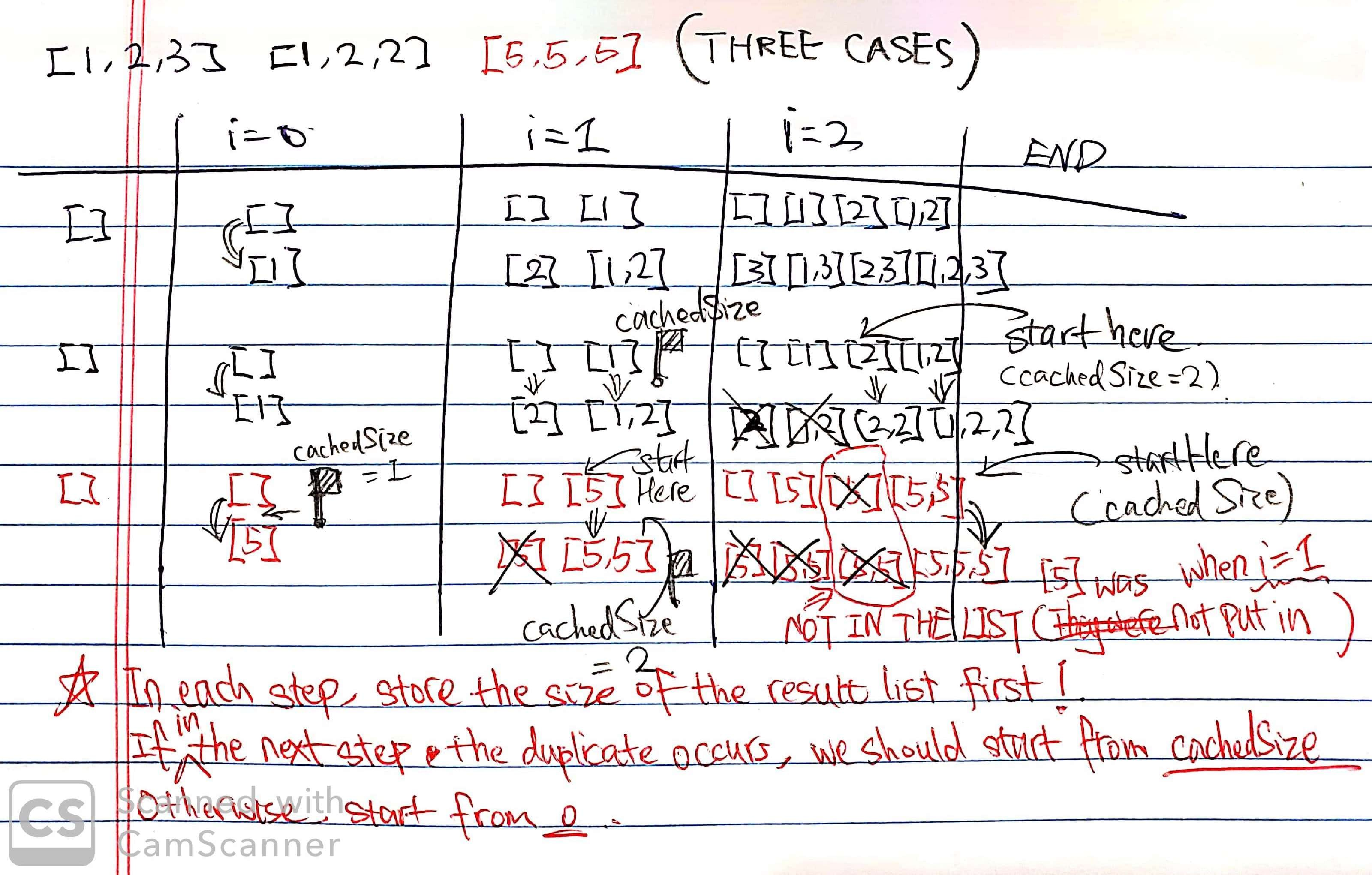# 78. Subsets I & II

Reference: Subsets I & Subsets II EPI 15.5
Difficulty: MediumI think it should be hard for Subsets II

## Problem

Given a set of distinct integers, nums, return all possible subsets (the power set).

Note: The solution set must not contain duplicate subsets.

Example:

Follow up: Check out the Subsets II section below.

## Analysis

The power set of a set $S$ is the set of all subsets of $S$, including both the empty set $\emptyset$ and $S$ itself. The power set of ${1, 2, 3}$ is graphically illustrated as follows.### Backtracking

The idea is that we loop over the number list. For each number, we have two choices: pick it, or not. For example, in [1, 2, 3], we pick $1$ and then do the same thing for the subproblem [2, 3]; and we don’t pick $1$ and then do the same thing for the subproblem [2, 3].

The size of subproblems is decreasing. When picking $2$, the subproblem becomes  instead of [1, 3].

Consider the following questions:

• What is the base case?
• When do we add the list to the result?

Here is an illustration of recursive process on [1, 2, 3].Note: Remember to add empty set manually.

Time: $O(N \times 2^N)$ since the recurrence is $T(N) = 2T(N - 1)$ and we also spend at most $O(N)$ time within a call.
Space: $O(N \times 2^N)$ since there are $2^N$ subsets. If we only print the result, we just need $O(N)$ space.

### Iteration

The idea is simple. We go through the elements in the nums list. For each element, we loop over the current result list we have constructed so far. For each list in the result, we make a copy of this list and append the current element to it (it means picking the element). It is based on the same idea in backtracking (in each step you have choices: pick or not pick).

The result list initially contains an empty list []. We loop over each element of nums, e.g. [1, 2, 3].

• After the first round, we have [[], ].
• After the second round, we have [[], , , [1,2]].
• After the third round, we have [[], , , [1,2], , [1,3], [2,3], [1,2,3]].

First, let’s go over an incorrect version. There are two errors:

1. We add a new element to L, but it changes the existed L. Thus, we should make a new copy of it.
2. While looping over result, we are modifying its size. In Java, the compiler would yell.

Incorrect version:

Correct version:

Time: $O(N\times 2^N)$

• The outer loop takes $O(N)$ time.
• The inner loop takes $2, 4, 8, \ldots, 2^N$ time respectively.
• In inner loop, making a new copy of L takes at most $O(N)$ time.
• Total runtime $T(N) = N \times (2 + 4 + 8 + \ldots + 2^N) \approx N \times 2^N$

Space: $O(N\times 2^N)$

### K-Size Subsets

Actually, we can use the code in 77. Combinations to solve this problem.

Time: $O(N\times 2^N)$

• $C^0_N + C^1_N + C^2_N + C^3_N + \ldots + C^N_N = 2^N$

Space: $O(N\times 2^N)$

## Subsets II Interesting!

Reference:

Given a collection of integers that might contain duplicates, nums, return all possible subsets (the power set).

Note: The solution set must not contain duplicate subsets.

### Backtracking

We need to know when we should not add a list to the result list.By observation, a duplicate list occurs when offset >= 1 (when offset = 0, duplicate cannot occur) and nums[offset - 1] == nums[offset] and in the previous step we did not pick nums[offset - 1]. The information of whether it picks or not could be passed down by a boolean parameter isPicked.

If the above condition is satisfied:

• Do not add the list to the result list.
• Do not do the subproblem after picking the current element.
• Only do the subproblem after not picking the current element.

Note: Be careful where we should put the numList.add(val) and numList.remove(numList.size() - 1).

Another version (similar):

Time: $O(N \times 2^N)$
Space: $O(N \times 2^N)$

### Iteration

Using the same idea in backtracking, we need to figure out when we should add a list to the result list. Check out three examples below ([1,2,3], [1,2,2], [5,5,5]).By observation, we learn that we should start from, if duplicate is detected, a specific location in the result list. In Subsets I, we always start from $0$.

Interestingly, the specific location corresponds to the initial size of the result list in the previous round. Since we change the result list in each round, we should cache the size of the result list as cachedSize.

Then we denote the starting index as startIdx. In each round, similar to what we’ve done in Subsets I, we set startIdx as:

• 0 (no duplicate or i == 0)
• cachedSize (duplicate occurs)

After setting startIdx, remember to do the caching job for the current size of the result list. Notice a fact that this cached size may not be used in the next round.

Time: $O(N \times 2^N)$
Space: $O(N \times 2^N)$

CommentJunhao Wang
a software engineering cat